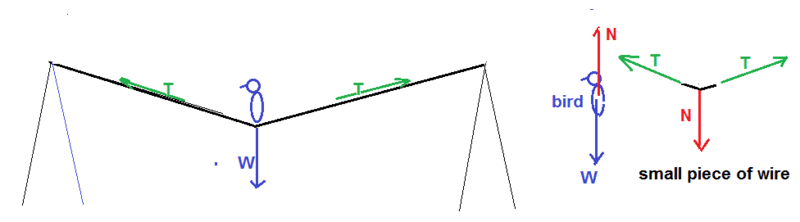# Bird on wire, tension

iluvfisics

## Homework Statement

0.5 kg bird lands on wire of telephone poles that are 50m apart. The wire sags 0.15m. What's the tension in the wire? Ignore weight of the wire

## The Attempt at a Solution

I figured out the angle that would be created (0.34 degrees) when the bird lands and when I go to make individual equations for the x and y components of the tension I put Fy=2Tsin(0.34) - W +N=0. When I check the answer they do not include the normal force (N) in the equation. Why is that? Is it because we are ignoring the weight of the wire?

mfb
Mentor
What are W and N?

iluvfisics
What are W and N?
W is weight (mg) and N is the normal force

mfb
Mentor
The normal force of what, and how does it differ from the weight?

iluvfisics
The normal force of what, and how does it differ from the weight?
The normal force meaning the force of the wire on the bird. Why would it not be included in the y-vector force equation?

Marc Rindermann
Gold Member
the normal force that counteracts the birds weight is provided by the wire. The weight only has a component in -y, the two forces along the wire to each side of the bird have a component each in the +y direction, the -x direction and the +x direction respectively. So the force components in the opposite x-directions cancel out each other and what's left of the tension in the wire needs to cancel out the weight of the bird.

it always helps to draw a force diagram to see which forces counteract which

haruspex
Homework Helper
Gold Member
2020 Award
The normal force meaning the force of the wire on the bird. Why would it not be included in the y-vector force equation?
First, define the system you are analysing: the bird, the wire, the combination, or whatever. Then consider all the forces on that system from outside it.
In the present case, to find the tension, you could consider the small segment of wire the bird sits on and the bird itself, as two separate systems related by their mutual normal forces. But simpler is to consider the combination of the two.
The forces on this are the tension each side on the wire segment and gravity on the bird. It does not include either normal force because they are internal to the system.

ehild
Homework Helper
First, define the system you are analysing: the bird, the wire, the combination, or whatever. Then consider all the forces on that system from outside it.
In the present case, to find the tension, you could consider the small segment of wire the bird sits on and the bird itself, as two separate systems related by their mutual normal forces. But simpler is to consider the combination of the two.
The forces on this are the tension each side on the wire segment and gravity on the bird. It does not include either normal force because they are internal to the system.
To @haruspex comment: see the picture.
Gravity and the normal force from the small piece of wire the bird sits on act on the bird. The normal force from the bird and the tensions in both pieces from the wire act on that small piece. Summing all forces exerted on the system bird+small piece of wire, the normal force cancel.•mfb and conscience